# Some inequalities for unitarily invariant norms of matrices

## Abstract

This article aims to discuss inequalities involving unitarily invariant norms. We obtain a refinement of the inequality shown by Zhan. Meanwhile, we give an improvement of the inequality presented by Bhatia and Kittaneh for the Hilbert-Schmidt norm.

### Mathematical Subject Classification

MSC (2010) 15A60; 47A30; 47B15

## 1. Introduction

Let M m,n be the space of m × n complex matrices and M n = M n,n . Let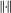denote any unitarily invariant norm on M n . So,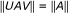for all AM n and for all unitary matrices U,VM n . For A = (a ij )M n , the Hilbert-Schmidt norm of A is defined by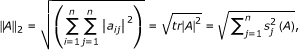where tr is the usual trace functional and s 1 (A) ≥ s 2 (A) ≥ ... ≥ s n-1 (A) ≥ s n (A) are the singular values of A, that is, the eigenvalues of the positive semidefinite matrix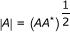, arranged in decreasing order and repeated according to multiplicity. The Hilbert-Schmidt norm is in the class of Schatten norms. For 1 ≤ p < , the Schatten p-norm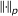is defined as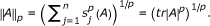For k = 1,...,n, the Ky Fan k-norm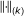is defined as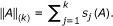It is known that these norms are unitarily invariant, and it is evident that each unitarily invariant norm is a symmetric guage function of singular values [1, p. 54-55].

Bhatia and Davis proved in  that if A,B,XM n such that A and B are positive semidefinite and if 0 ≤ r ≤ 1, then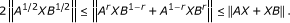(1.1)

Let A,B,XM n such that A and B are positive semidefinite. In , Zhan proved that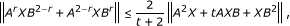(1.2)

for any unitarily invariant norm and real numbers r,t satisfying 1 ≤ 2r ≤ 3,-2 < t ≤ 2. The case r = 1,t = 0 of this result is the well-known arithmetic-geometric mean inequality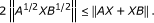Meanwhile, for r[0,1], Zhan pointed out that he can get another proof of the following well-known Heinz inequality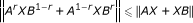by the same method used in the proof of (1.2).

Let A,B,XM n such that A and B are positive semidefinite and suppose that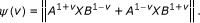(1.3)

Then ψ is a convex function on [-1,1] and attains its minimum at v = 0 [4, p. 265].

In , for positive semidefinite n × n matrices, the inequality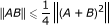(1.4)

was shown to hold for every unitarily invariant norm. Meanwhile, Bhatia and Kittaneh  asked the following.

### Question

Let A,BM n be positive semidefinite. Is it true that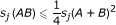,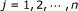?

The case n = 2 is known to be true . (See also, [1, p. 133], [6, p. 2189-2190], [7, p. 198].)

Obviously, if A,BM n are positive semidefinite and AB = BA, then we have,.

## 2. Some inequalities for unitarily invariant norms

In this section, we first utilize the convexity of the function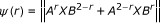to obtain an inequality for unitarily invariant norms that leads to a refinement of the inequality (1.2). To do this, we need the following lemmas on convex functions.

### Lemma 2.1

Let A,B,XM n such that A and B are positive semidefinite. Then, for each unitarily invariant norm, the functionis convex on [0,2] and attains its minimum at r = 1.

### Proof

Replace v+1 by r in (1.3).□

### Lemma 2.2

Let ψ be a real valued convex function on an interval [a,b] which contains (x1,x2). Then for x1xx2, we have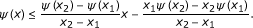(2.1)

### Proof

Since ψ is a convex function on [a,b], for ax1xx2b, we have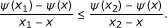This is equivalent to the inequality (2.1).□

### Theorem 2.1

Let A,B,XM n such that A and B are positive semidefinite. If 1 ≤ 2r ≤3 and -2 <t ≤ 2, then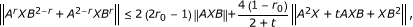(2.2)

where r0 = min{r,2-r}.

### Proof

If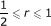, then by Lemma 2.1 and Lemma 2.2, we have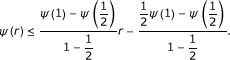That is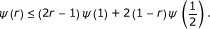(2.3)

It follows from (1.2) and (2.3) that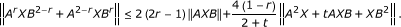If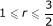, then by Lemma 2.1 and Lemma 2.2, we have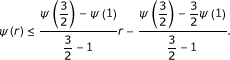That is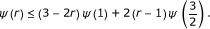(2.4)

It follows from (1.2) and (2.4) that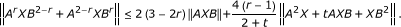It is equivalent to the following inequality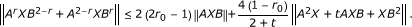This completes the proof.□

Now, we give a simple comparison between the upper bound in (1.2) and the upper bound in (2.2).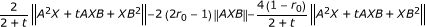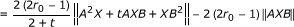Therefore, Theorem 2.1 is a refinement of the inequality (1.2).

Let A,B,XM n such that A and B are positive semidefinite. Then, for each unitarily invariant norm, the function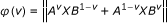is a continuous convex function on [0,1] and attains its minimum at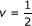. See [4, p. 265]. Then, by the same method above, we have the following result.

### Theorem 2.2.

Let A,B,XM n such that A and B are positive semidefinite. If 0 ≤ v ≤ 1, then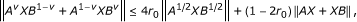where r0 = min{v,1-v}. This is a refinement of the second inequality in (1.1).

Next, we will obtain an improvement of the inequality (1.4) for the Hilbert-Schmidt norm. To do this, we need the following lemma.

### Lemma 2.3.

Let A,B,XM n such that A and B are positive semidefinite. If 0 ≤ v ≤ 1, then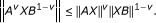### Theorem 2.3

Let A,B,XM n such that A and B are positive semidefinite. If 0 ≤ v ≤ 1, then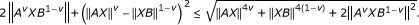### Proof

Let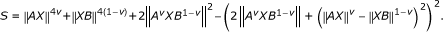So,By Lemma 2.3, we have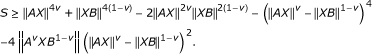That is,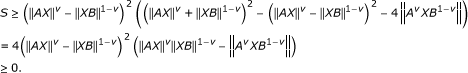Hence,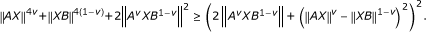This completes the proof.□

Let A,B,XM n such that A and B are positive semidefinite, for Hilbert-Schmidt norm, the following equality holds: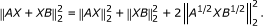Takingin Theorem 2.3, and then we have the following result.

### Theorem 2.4.

Let A,B,XM n such that A and B are positive semidefinite. Then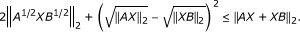Bhatia and Kittaneh proved in  that if A,BM n are positive semidefinite, then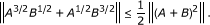(2.5)

Now, we give an improvement of the inequality (1.4) for the Hilbert-Schmidt norm.

### Theorem 2.5

Let A,BM n be positive semidefinite. Then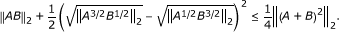### Proof

Let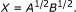Then, by Theorem 2.4, we have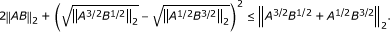(2.6)

It follows form (2.5) and (2.6) that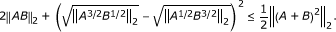That is,This completes the proof.□

## References

1. Zhan X: Matrix Theory. Higher Education Press, Beijing; 2008.

2. Bhatia R, Davis C: More matrix forms of the arithmetic-geometric mean inequality. SIAM J Matrix Anal Appl 1993, 14: 132–136. 10.1137/0614012

3. Zhan X: Inequalities for unitarily invariant norms. SIAM J Matrix Anal Appl 1998, 20: 466–470. 10.1137/S0895479898323823

4. Bhatia R: Matrix Analysis. Springer-Verlag, New York; 1997.

5. Bhatia R, Kittaneh F: Notes on matrix arithmetic-geometric mean inequalities. Linear Algebra Appl 2000, 308: 203–211. 10.1016/S0024-3795(00)00048-3

6. Bhatia R, Kittaneh F: The matrix arithmetic-geometric mean inequality revisited. Linear Algebra Appl 2008, 428: 2177–2191.

7. Bhatia R: Positive Definite Matrices. Princeton University Press, Princeton; 2007.

8. Kittaneh F: On the convexity of the Heinz means. Integr Equ Oper Theory 2010, 68: 519–527. 10.1007/s00020-010-1807-6

9. Kittaneh F: Norm inequalities for fractional powers of positive operators. Lett Math Phys 1993, 27: 279–285. 10.1007/BF00777375

10. Kittaneh F, Manasrah Y: Improved Young and Heinz inequalities for matrices. J Math Anal Appl 2010, 361: 262–269. 10.1016/j.jmaa.2009.08.059

## Acknowledgements

The authors wish to express their heartfelt thanks to the referees and Professor Vijay Gupta for their detailed and helpful suggestions for revising the manuscript. At the same time, we are grateful for the suggestions of Yang Peng. This research was supported by Natural Science Foundation Project of Chongqing Science and Technology Commission (No. CSTC, 2010BB0314), Natural Science Foundation of Chongqing Municipal Education Commission (No. KJ101108), and Scientific Research Project of Chongqing Three Gorges University (No. 10ZD-16).

## Author information

Authors

### Corresponding author

Correspondence to Limin Zou.

### Competing interests

The authors declare that they have no competing interests.

### Authors' contributions

SW and LZ designed and performed all the steps of proof in this research and also wrote the paper. YJ participated in the design of the study and suggest many good ideas that made this paper possible and helped to draft the first manuscript. All authors read and approved the final manuscript.

## Rights and permissions

Reprints and Permissions

Wang, S., Zou, L. & Jiang, Y. Some inequalities for unitarily invariant norms of matrices. J Inequal Appl 2011, 10 (2011). https://doi.org/10.1186/1029-242X-2011-10